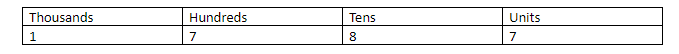QuestionAnswers

# Fill in the blanks:The unit digit of the cube of the number 1787 is …………………….Verified
128.4k+ views
Hint: Any number which has 7 in the units place, when we take the cube of that number then the digits in the units place will be the same as the digit in the units place of ${7^3}$.

According to the question, we need to find the unit digit in the cube of the number 1787 . Now the digit in the units place in 1787 is 7. Let us first place the digits of 1787 as per the decimal system:Now, we have 7 in the unit's place in 1787.
So the cube of 1787 means multiplying 1787 with itself thrice, that is,${\left( {1787} \right)^3} = \left( {1787} \right) \times \left( {1787} \right) \times \left( {1787} \right)$ .
So, clearly the digit in the units place being 7 is also being cubed, which means that the digit in the units place in the cube of 1787 will be the same as the digit in the units place in the cube of 7.
So, ${7^3} = 7 \times 7 \times 7 = 343$ .
Thus, the digit in the units place of ${7^3}$ is 3
$\Rightarrow$ the digit at the units place of ${\left( {1787} \right)^3}$ will be 3.
Hence, the unit digit of the cube of the number 1787 is …..3…..

Note: The unit digit of any number raised to the power of ‘n’ depends on the unit digit of the original number. However the unit digit keeps changing only till a number is raised to a maximum power of 4. After that the unit digit starts repeating itself. For example let us consider a number 43.
So, ${\left( {43} \right)^1}$ has $3 \times 1 = 3$ as its unit digit.
${\left( {43} \right)^2}$ has $3 \times 3 = 9$ as its unit digit.
${\left( {43} \right)^3}$ has $3 \times 3 \times 3 = 27$,that is 7 as its unit digit.
${\left( {43} \right)^4}$ has $3 \times 3 \times 3 \times 3 = 81$, that is 1 as its unit digit.
${\left( {43} \right)^5}$, has $3 \times 3 \times 3 \times 3 \times 3 = 243$, that is 3 as its unit digit.
So, as soon as the power increases by 1 from 4 and becomes 5, the unit digit also starts repeating itself from 3 onwards.